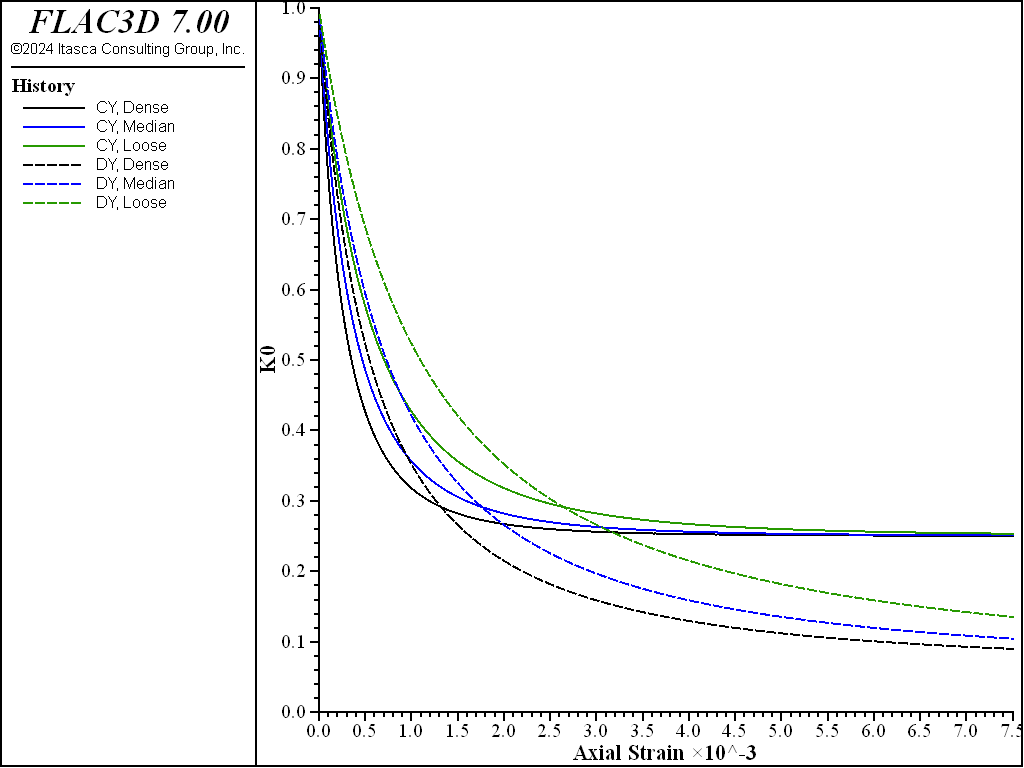FLAC3D Theory and Background • Constitutive Models

# Oedometer Test with CYSoil Model

Note

To view this project in FLAC3D, use the menu command Help ► Examples…. Choose “ConstitutiveModels/ OedometerCYSoil” and select “OedometerCYSoil.prj” to load. The project’s main data file is shown at the end of this example.

Oedometer test simulations are carried out using the CYSoil model for different values of the parameter $$\alpha$$ to evaluate the impact of the cap aspect ratio on confining stress when yielding occurs on the cap. The values of $$\alpha$$ considered for the tests are 0.5, 1, 1.5, and 1020 (i.e., a value that is very large compared to 1, in which case the behavior of the double-yield model is recovered).

We use the same setup and properties (apart from $$\alpha$$) as in the isotropic compression example, except in this case, the simulations are run in plane strain, with fixed lateral boundaries to simulate oedometer test conditions. Friction is assigned a large value to prevent shear yielding.

The stress ratio, $$K_0$$, of confining stress to vertical stress, $$\sigma_{xx}$$ / $$\sigma_{yy}$$, is plotted versus axial strain for dense, medium, and loose sand in three figures: Figure 1 compares predictions for $$\alpha$$ = 0.5 and $$\alpha$$ = $$10^{20}$$ (double-yield model); Figure 2 compares predictions for $$\alpha$$ = 1.0 and $$\alpha$$ = $$10^{20}$$ (double-yield model); and Figure 3 shows plots for $$\alpha$$ = 1.5 and $$\alpha$$ = $$10^{20}$$ (double-yield model).

The results of the oedometer simulations show that, with the given properties, a higher value of $$K_0$$ is achieved for the CYSoil model than the double-yield model. And for all tests, the lower the aspect ratio of the cap, the higher the $$K_0$$ that is achieved. Also, dense, medium, and loose sands converge to the same ultimate $$K_0$$ value as deformation takes place, and they do so at a faster deformation rate for the CYSoil model than the double-yield model.Figure 1: $$K_0$$ versus axial strain for dense, medium, and loose sand—$$\alpha$$ = 0.5 (solid lines) and double-yield model (dashed lines).Figure 2: $$K_0$$ versus axial strain for dense, medium, and loose sand— $$\alpha$$ = 1.0 (solid lines) and double-yield model (dashed lines).Figure 3: $$K_0$$ versus axial strain for dense, medium, and loose sand— $$\alpha$$ = 1.5 (solid lines) and double-yield model (dashed lines).

Data File

OedometerCYSoil.dat

model new
model title "Oedometer test - dense, medium, loose sand - CYSoil and DY"
model large-strain off
;
zone create brick size 3 1 5
zone group 'gcy'   range position-x 0 1
zone group 'gdy'   range position-x 2 3
zone group 'gnull' range union position-x 1 2 position-z 1 2 position-z 3 4
;
[global _pc0   = 10.]
;
zone cmodel assign cap-yield range group 'gcy'
zone property density=1000 pressure-reference=100. poisson=0.2 ...
multiplier=[2.0/3.0] flag-cap=1 flag-shear=1 friction=89.0  ...
pressure-cap=[_pc0]  pressure-initial=[_pc0]  range group 'gcy'
;
zone cmodel double-yield range group 'gdy'
zone property density=1000 bulk-maximum=1.e15 shear-maximum=0.75e15 ...
friction=89.0 pressure-cap=[_pc0] multiplier=[2.0/3.0] ...
range group 'gdy'
;
zone cmodel assign null range group 'gnull'
;
program call 'fish' suppress
; double-yield
[cap_table('1', 300.)]
zone property strain-volumetric-plastic=[evp0] table-pressure-cap='1' ...
range group 'gdy' position-z 4 5
[cap_table('2', 225.)]
zone property strain-volumetric-plastic=[evp0] table-pressure-cap='2' ...
range group 'gdy' position-z 2 3
[cap_table('3', 150.)]
zone property strain-volumetric-plastic=[evp0] table-pressure-cap='3' ...
range group 'gdy' position-z 0 1
; cap-yield-soil
zone property shear-reference 300. range group 'gcy' position-z 4 5
zone property shear-reference 225. range group 'gcy' position-z 2 3
zone property shear-reference 150. range group 'gcy' position-z 0 1
;
zone gridpoint fix velocity
zone gridpoint initialize velocity-z -1.25e-7 ...
range union position-z 1 position-z 3 position-z 5
zone initialize stress xx [-_pc0] yy [-_pc0] zz [-_pc0]
;
[_hist_setup]
history interval 100
zone history displacement-z position (0,0,1)
fish history _k0_d_cy
fish history _k0_m_cy
fish history _k0_l_cy
fish history _k0_d_dy
fish history _k0_m_dy
fish history _k0_l_dy
model save 'ini'
; -- alpha = 0.5
zone property alpha=0.5 range group 'gcy'
model step 60000
model save 'al1'
; -- alpha = 1.0
model restore 'ini'
zone property alpha=1.0 range group 'gcy'
model step 60000
model save 'al2'
; -- alpha = 1.5
model restore 'ini'
zone property alpha=1.5 range group 'gcy'
model step 60000
model save 'al3'